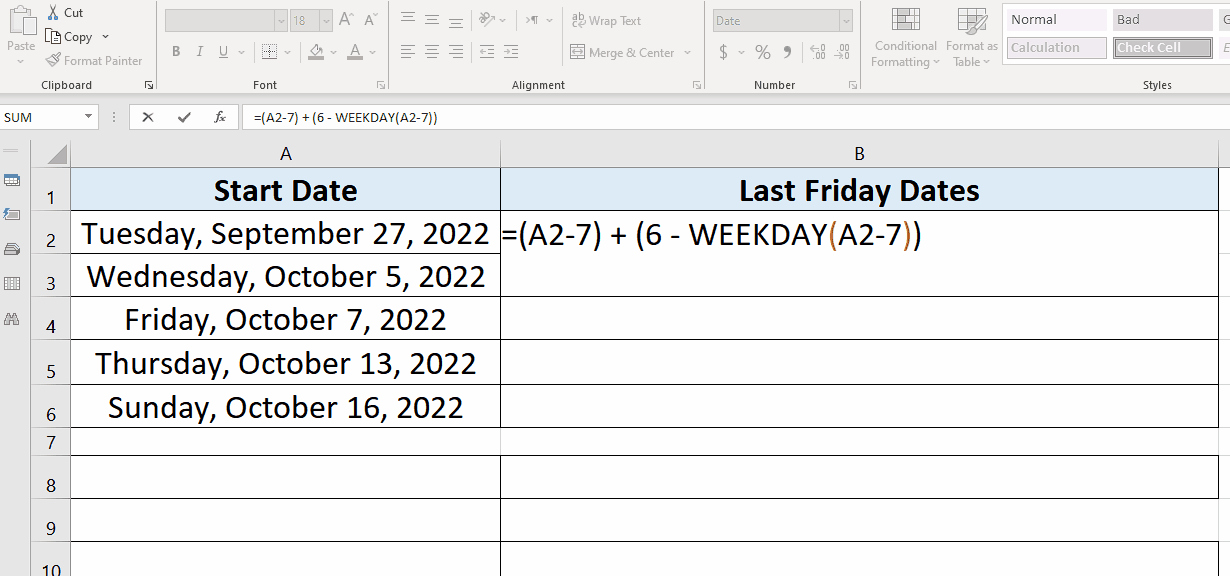# How to get the last Friday date in Excel

In this tutorial we’ll learn how to find last Friday (i.e. The Friday of the last week) from any given date. Let’s look at the data set in the picture above. We have a column with start dates and we’ll find the last Friday from all these dates. Let’s do this by following these steps;While working with dates in Excel, it is often required to find a specific day from a particular date. Excel has some built-in formulas to handle the addition and subtraction of dates. However, the formula to find a particular day from a defined date is not straightforwardly available in Excel.

### Step 1 – Create the appropriate formula– To find a specific day from any given date, we’ll have to use the WEEKDAY() function.

– The required formula will be as follows;

(date – 7) + (6 – WEEKDAY(date – 7))

date: This will be the date from which we wish to find the last Friday date. In our case it will be cell A2.

– Write the above mentioned formula into the appropriate cell as shown in the figure.

### Step 2 – Implement the formula to get last Friday Date– After writing the formula, just press enter and you will get the last Friday date in the cell.

– With the data set, we have shown the snapshots of the calendar as well. This is placed with the data set to compare the results of the formula with actual dates.

## Breakdown of the formula:

We have used the WEEKDAY function in the following format;

(date – 7) + (6 – WEEKDAY(date – 7))

Excel has assigned each day of the week a unique number and each day is identified by that number. In the default settings of Excel, the week starts from Sunday (so the number for Sunday is 1) and ends on Saturday (the number of Saturday is 7). When any date is passed to the WEEKDAY() function, it returns a number between 1 ~ 7 depending upon the day of the week on that particular date.

So our formula jumps to the last weekday and then counts to the correct date by using the calculations with WEEKDAY() function. In our formula the last 6 represent Friday. If we change that 6 to any other number then we can get that day of the week by using the same formula.